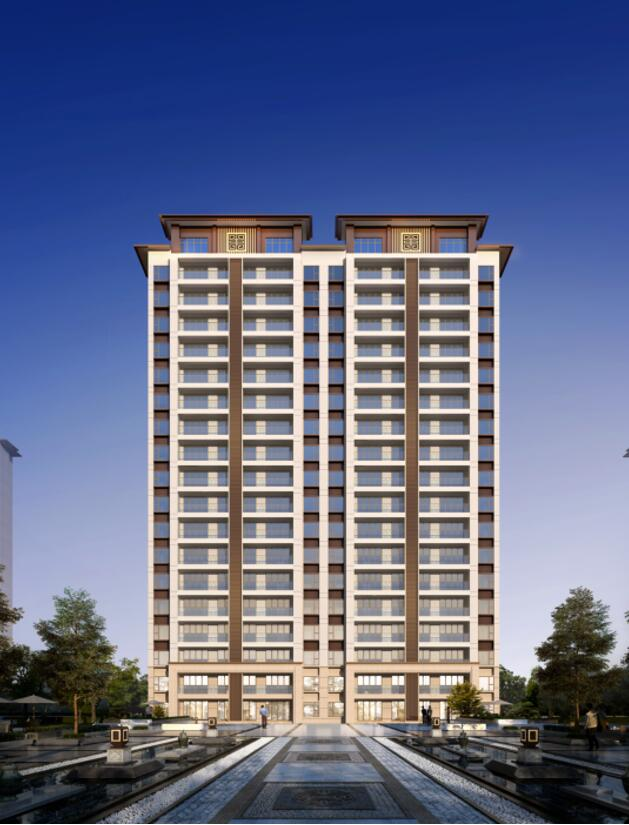## 网站地图## 碧桂园·黄金时代（濮阳）

### 濮阳

#### 地址

• 资阳
• 本溪
• 北流
• 万载
• 慈溪
• 长春
• 通化
• 西昌
• 丰城
• 阳春
• 鹤山
• 江油
• 辛集
• 辽源
• 衡水
• 黔西南州
• 黔东南州
• 石家庄
• 唐山
• 秦皇岛
• 邯郸
• 邢台
• 保定
• 张家口
• 承德
• 沧州
• 廊坊
• 黄骅
• 迁安
• 怀来
• 洛阳
• 驻马店
• 郑州
• 开封
• 平顶山
• 安阳
• 鹤壁
• 新乡
• 焦作
• 濮阳
• 许昌
• 漯河
• 三门峡
• 南阳
• 商丘
• 信阳
• 周口
• 武汉
• 黄石
• 十堰
• 宜昌
• 襄阳
• 荆州
• 荆门
• 鄂州
• 仙桃
• 潜江
• 黄冈
• 随州
• 咸宁
• 恩施
• 怀化
• 长沙
• 株洲
• 湘潭
• 湘西州
• 张家界
• 益阳
• 郴州
• 衡阳
• 岳阳
• 常德
• 娄底
• 徐州
• 南京
• 无锡
• 镇江
• 泰州
• 淮安
• 舟山
• 黔西南布依族苗族自治州
• 临沂
• 凉山
• 普洱
• 红河
• 锦州
• 葫芦岛
• 东方
• 咸阳
• 丽水
• 定安县
• 吕梁
• 来宾
• 河池
• 宜宾
• 重庆市
• 雅安
• 潮州
• 大理
• 巴中
• 桂林
• 贵港
• 百色
• 柳州
• 贺州
• 儋州
• 福清
• 威海
• 烟台
• 乐山
• 金华
• 永泰
• 潍坊
• 滨州
• 德州
• 临汾
• 日照
• 眉山
• 武威
• 乌鲁木齐
• 保山
• 临沧
• 防城港
• 达州
• 遂宁
• 绵阳
• 淮南
• 宝鸡
• 枣庄
• 桂平
• 景德镇
• 临夏
• 聊城
• 崇左
• 马鞍山
• 安顺
• 北海
• 菏泽
• 铜陵
• 黔南州
• 蚌埠
• 嘉兴
• 淮北
• 都匀
• 南宁
• 兴义
• 南平
• 松溪
• 吉安
• 大同
• 陆丰
• 厦门
• 铜仁
• 银川
• 昭通
• 包头
• 遵化
• 漳州
• 深圳
• 霸州
• 香港
• 合肥
• 抚州
• 琼海
• 定西
• 南昌
• 宿州
• 文昌
• 临高县
• 陵水
• 海口
• 博鳌
• 三亚
• 绥化
• 哈尔滨
• 杭州
• 绍兴
• 宁波
• 温州
• 衢州
• 湖州
• 台州
• 天津
• 沈阳
• 大连
• 通辽
• 兴安盟
• 呼伦贝尔
• 运城
• 晋中
• 太原
• 南通
• 宿迁
• 扬州
• 盐城
• 连云港
• 苏州
• 常州
• 安庆
• 黄山
• 巢湖
• 芜湖
• 池州
• 宣城
• 六安
• 古田
• 无为
• 桐城
• 亳州
• 南陵
• 庐江
• 阜阳
• 滁州
• 孝感
• 天门
• 邵阳
• 永州
• 平果
• 玉林
• 梧州
• 钦州
• 梅州
• 河源
• 云浮
• 东莞
• 汕头
• 中山
• 清远
• 惠州
• 韶关
• 肇庆
• 阳江
• 汕尾
• 茂名
• 江门
• 佛山
• 广州
• 雷州
• 英德
• 湛江
• 揭阳
• 珠海
• 信宜
• 济南
• 淄博
• 青岛
• 泰安
• 济宁
• 马来西亚雪兰莪州
• 马来西亚柔佛州
• 永春
• 南安
• 三明
• 宁德
• 武夷山
• 福鼎
• 莆田
• 建瓯
• 漳浦
• 福安
• 福州
• 泉州
• 龙岩
• 兰州
• 张掖
• 天水
• 白银
• 贵阳
• 仁怀
• 毕节
• 凯里
• 六盘水
• 遵义
• 广元
• 德阳
• 南充
• 泸州
• 攀枝花
• 广安
• 成都
• 宜春
• 萍乡
• 九江
• 赣州
• 上饶
• 曲靖
• 楚雄
• 昆明
• 白城
• 北京
• 澳洲莱德
• 西安
• 渭南
• 上海
• 西宁（1）教育配套：项目紧邻艾菲国际幼儿园、濮阳市油田十八中、建设中的濮阳市公立幸福双语幼儿园以及在建油田第五小学分校；油田五小是濮阳市重点小学，学校先后被命名为全国青少年素质教育实践基地、河南省德育实验学校、河南省现代技术教育实验学校等荣誉，为业主们提供最优质的教育资源，为您的孩子提供一站式的教育服务，满足各学龄段孩子的教育需求，解决家长们的后顾之忧。

（2）医疗配套：距离项目不足1.5公里有濮阳市中医院，该院始建于1987年9月，是国家三级甲等中医院，河南中医药大学附属医院；距离项目15分车程的濮阳油田总医院，作为豫北区域的著名综合性三级甲等医院，是一家集医疗、教学、科研、急救、康复、预防保健于一体的集团化医疗机构，隶属于中华医疗集团。由此可见整个区域的医疗配套非常完善，为区域的发展提供强有力的医疗保障。

（3）生活配套：靠近市中心庆路往北，便是濮阳市最为成熟的商业圈，银座、丹尼斯、万嘉360等大型百货商场，同时项目周边基础生活配套齐全，可以满足各项生活需求。

（4）生态价值：（濮阳市植物园）1.电梯两侧开门，尽享私密，电梯厅优化利用利用

2.南北超大双阳台，真通透，面积合理计算

3.四开间朝南，享受充足的阳光

4.南向卧室全部附带飘窗，不计面积

5.南向客厅开间约4.8米，超大开间，阳光满溢，宽敞明亮

1.电梯两侧开门，入户电梯厅，尽享私密，归家的仪仗

2.四开间朝南，享受充足的阳光

3.卧室全部附带飘窗，不计面积

4.南向老人房，带卫生间，给老人舒适的晚年生活

5.空中花园 养花种菜皆宜

6.南向客厅开间约5.1米，超大开间，尽显主人尊贵奢华

7.南北双阳台，南北通透

1.电梯两侧开门，尽享私密，电梯厅利用率高

2.南侧超大阳台，真通透，面积合理计算

3.三开间朝南，享受充足的阳光

4.南向卧室全部附带飘窗

5.户型方正、动静分离